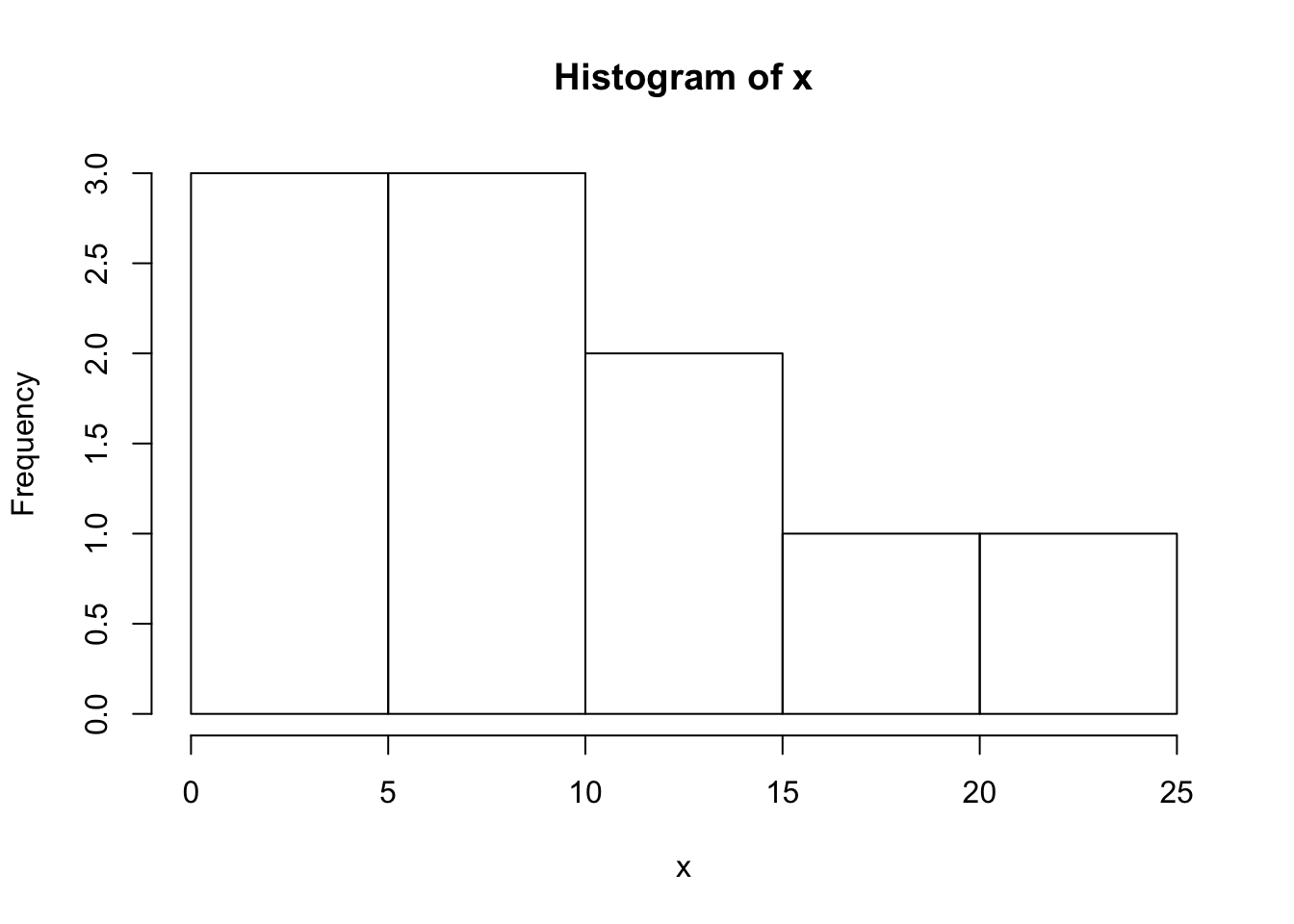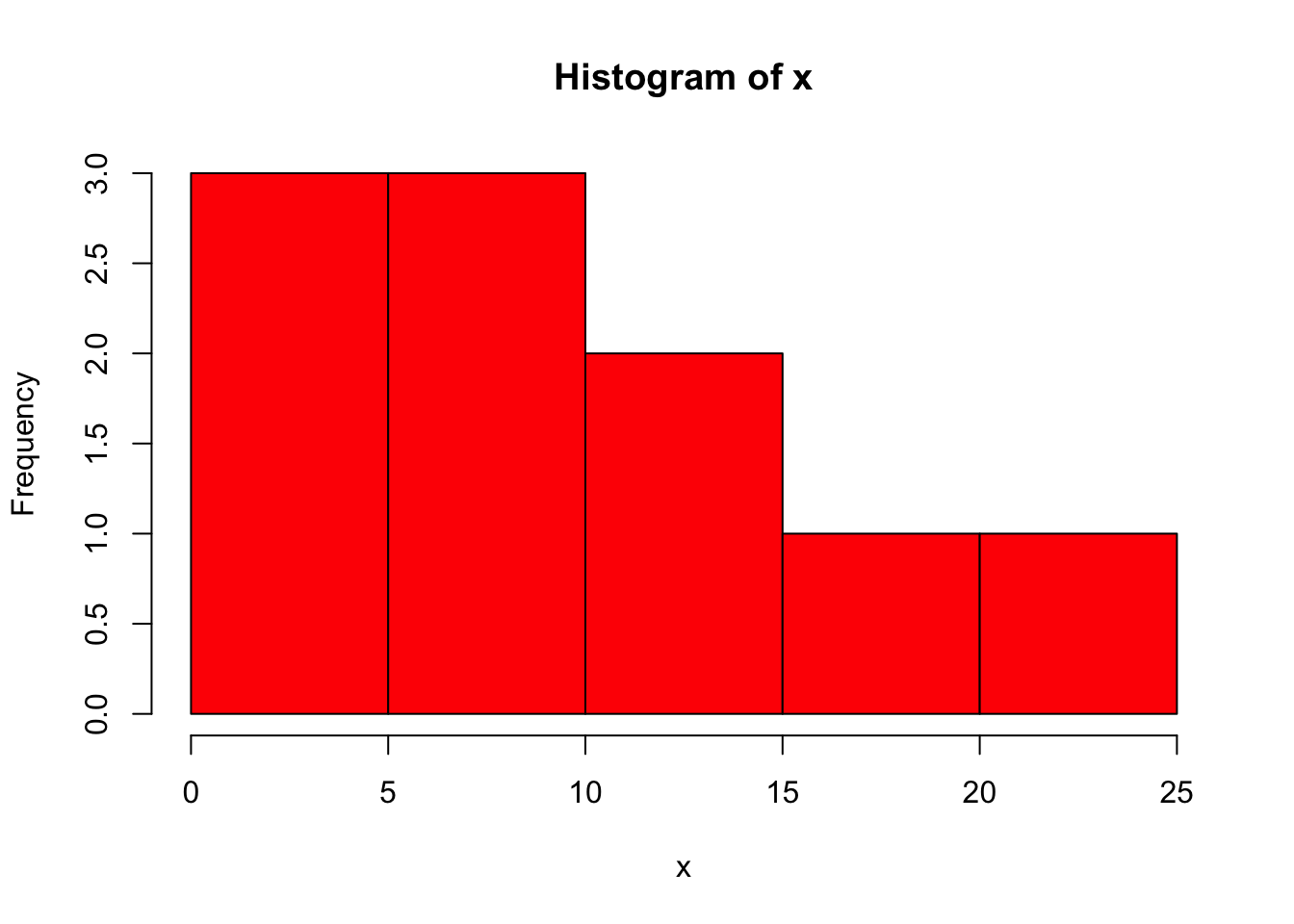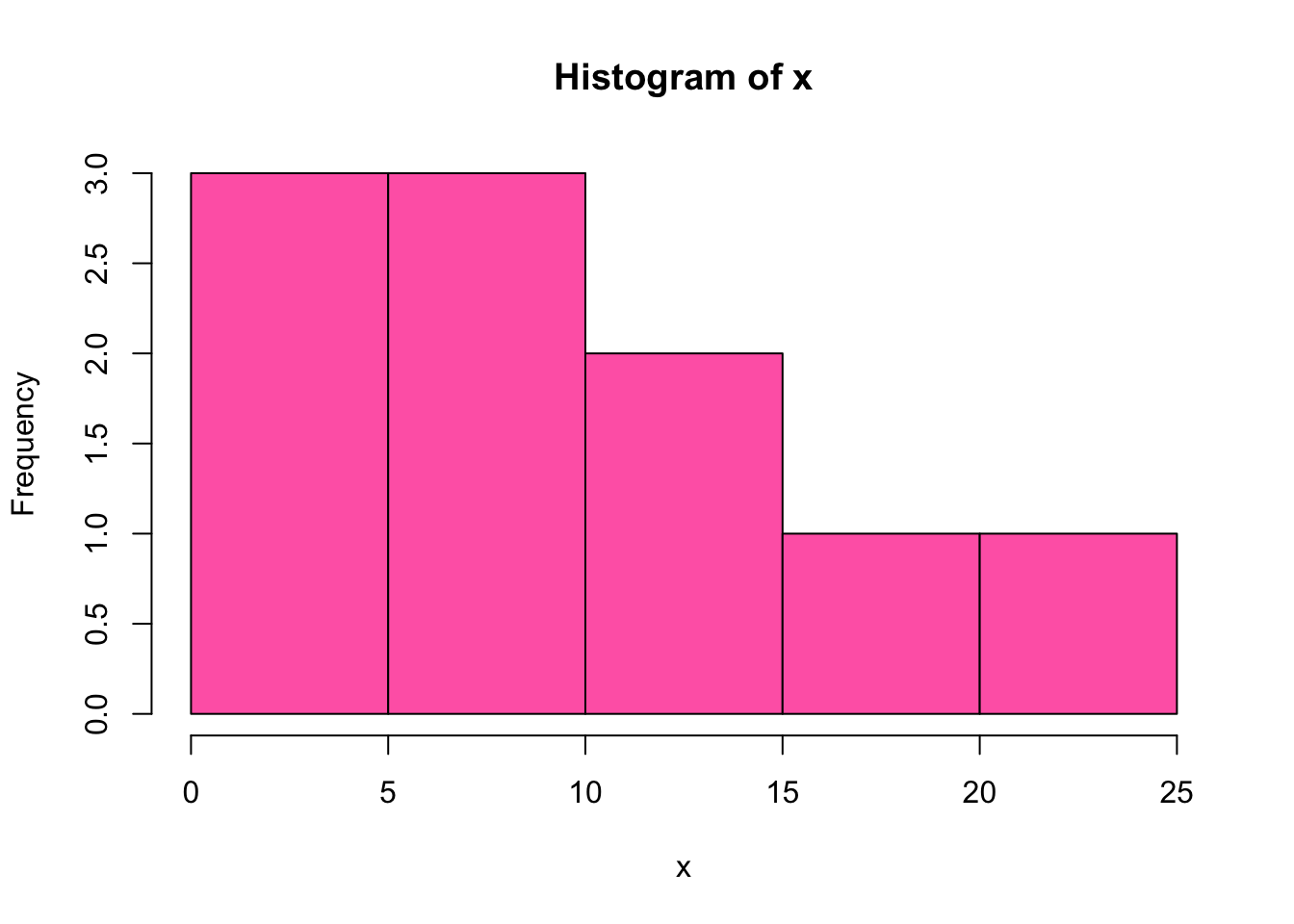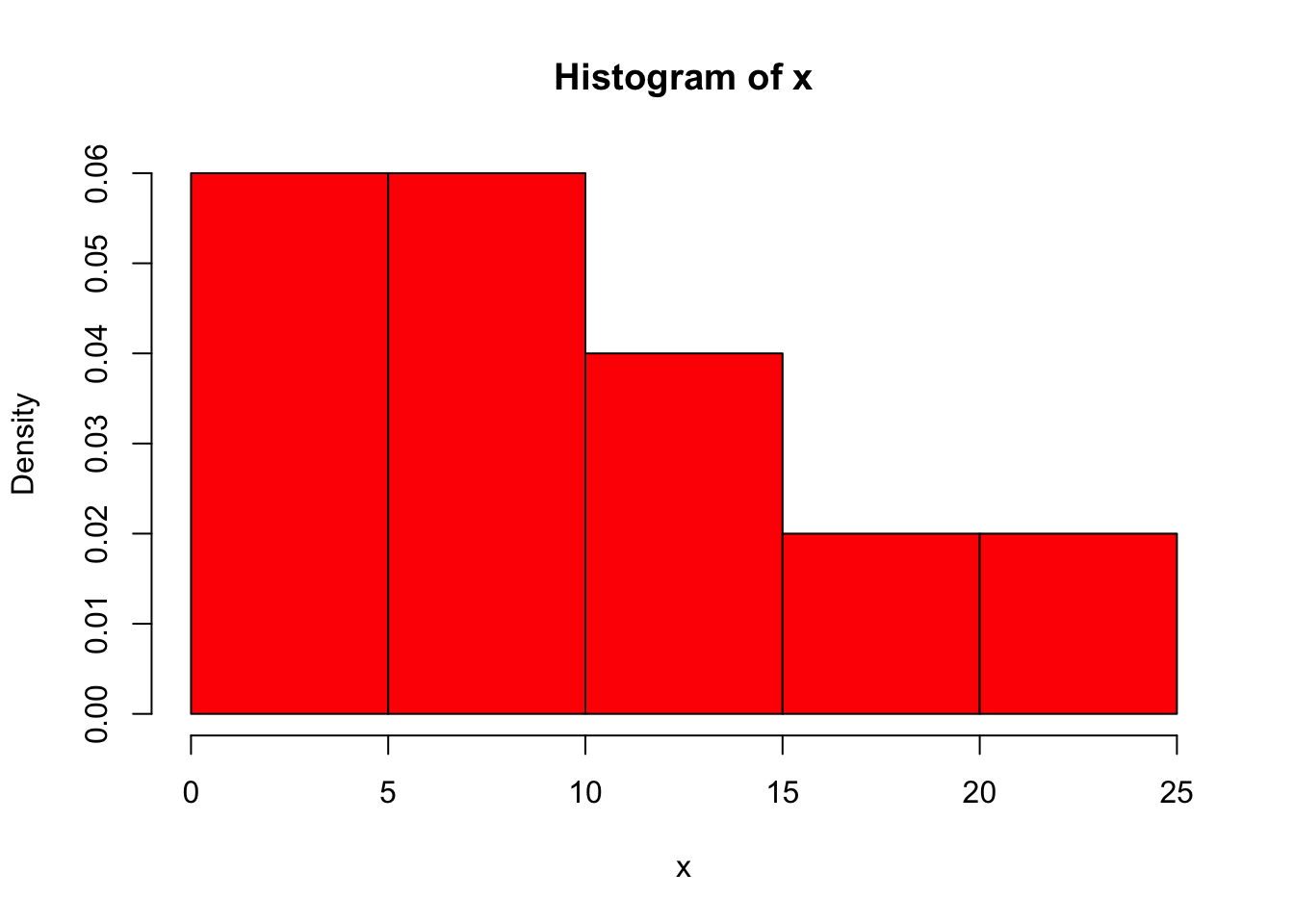## Basics of R: variables, vectors and descriptive statistics

### R as a calculator

Let’s look at very basic calculations in R.

``1 + 4 # addition``
``##  5``
``4 - 9 # subtraction``
``##  -5``
``6 * 5 + 7 / 2 # multiplication and division``
``##  33.5``
``sqrt(36) # taking a square root``
``##  6``
``6 ^ 2 # raising to power``
``##  36``
``6 ** 2 # the same``
``##  36``

Note: if you are planning to work both in R and Python, you had better memorize the latter variant of raising a number to some power (via `**`) since in Python the operator `^` corresponds to the bitwise addition that has nothing in common with powers.

In R we can calculate logarithms as well. By default the `log()` function returns the natural logarithm, the logarithm of the base `e`. In English books it is usually denoted as `log`, in Russian ones it is denoted as `ln`.

``log(4)``
``##  1.386294``

We can also specify the base of a logarithm adding the option `base`:

``log(4, base = 2)  # so 2^2 = 4``
``##  2``

Or calculate a logarithm of a base 10:

``log10(100)  # the same as log(100, base=10)``
``##  2``

If we want to round the results obtained, we can use the function `round()`:

``round(12.57)``
``##  13``

By default it rounds a value to the closest integer, so we got 13 above. However, we can specify the number of digits we want to see after a decimal point:

``round(12.57, 1)  # round to tenths, 1 digit after .``
``##  12.6``

### Variables in R

Names of the variables in R can contain letters, numbers, dots and underscores, but the name of a variable cannot start with a number (as in many programming languages). A name of a variable should not coincide with the reserved R words and operators (like `if`, `else`, `for`, `while`, etc).

Both operators `<-` and `=` can be used for assigning values to variables, but `<-` is a ‘canonical’ R operator that is usually applied in practice. In other words, writing code with `=` is technically correct, but not cool and has to be avoided :)

``````a <- 3
a``````
``##  3``

We can change the value of a variable and save it again with the same name:

``````x <- 2
x <- x + 3
x # updated, now it is 3 + 2 = 5``````
``##  5``

We can also assign text values to variables. A text is usually written in quotes:

``````s <- "hello"
s ``````
``##  "hello"``

It does not matter which quotes, single `''` or double `""` we will use. The only important thing is that the opening and the closing quote should be of the same type, so it is not allowed to write something like this: `"hello'`.

There are many functions that are aimed at working with text variables (in R they are called character variables), but now we will not concentrate on them. Just as an example, look at the function `toupper()` that converts all letters into capital ones:

``toupper(s)``
``##  "HELLO"``

Note that the original value of `s` has not changed, it is still in small letters:

``s``
``##  "hello"``

To save changes we have to reassign a value to `s`:

``````s <- toupper(s)
s  # updated``````
``##  "HELLO"``

### Vectors in R

A vector in R is a list (a series) of elements. It is created in the following way using the special function `c()`:

``v <- c(1, 0, 0, 1, 2) # vector v``

We can look at this vector:

``v``
``##  1 0 0 1 2``

To get the type of a vector (at least, whether it is numeric or not), we can use the function `class()`:

``class(v) # numeric values, not text ones``
``##  "numeric"``

Also we can define a length of a vector, i.e. a number of its elements:

``length(v)``
``##  5``

So as to choose an element of a vector by its index (its position in a vector), we should specify it in square brackets:

``v  # first element``
``##  1``
``v  # second element ``
``##  0``

Note that in R the numeration starts from 1, so if you got used to Python or other programming languages, take this into account. Requesting a zero element will result in nothing:

``v  # no error, but no such element``
``## numeric(0)``

Not only numeric vectors can be created, character ones are possible:

``````names <- c('Ann', 'Tom')
names``````
``##  "Ann" "Tom"``

### Descriptive statistics in R

Consider the following sample (we save its elements to the vector `x`):

``````x <- c(6, 6, 7, 0, 14, 24, 16, 15, 2, 0)
x``````
``##    6  6  7  0 14 24 16 15  2  0``

Let’s calculate several descriptive statistics for a numeric sample.

``min(x) # maximum value``
``##  0``
``max(x) # maximum value``
``##  24``
``mean(x) # an average, a sample mean``
``##  9``
``median(x) # a median``
``##  6.5``
``var(x) # a sample variance``
``##  63.11111``
``sd(x) # a standard deviation``
``##  7.94425``

Note: by default R computes a corrected sample variance (with good statistical properties), one with \(n-1\) in the denominator.

And what if we work with a categorical sample? For example, we have a text (character) vector:

``y <- c("a", "b", "c", "a", "c", "c")``

We can calculate the frequences of the values using the fucntion `table()`:

``table(y)``
``````## y
## a b c
## 2 1 3``````

This function returns absolute frequences. To get relative ones, we can compute them manually dividing every absolute frequency by the sum of all frequences for a sample:

``table(y)/sum(table(y))``
``````## y
##         a         b         c
## 0.3333333 0.1666667 0.5000000``````

Now let us proceed to histograms (of course, it is suitable only for numeric vectors). We can plot a histogram of our sample `x`:

``hist(x)  # hist - from histogram``By default a histogram is white, but you can add a color:

``hist(x, col="red")  # col for color``Or:

``hist(x, col="hotpink")  # more interesting color``There is a lot of colors in R, see the full list here.

Now we will not focus on styling, we will discuss it later, but we should mention two points important from a statistical standpoint: setting different types of values by a vertical axis and choosing a different number of bins (rectangles in a histogram).

We can indicate normalised frequences by a vertical axis, i.e. values adjusted in such a way that a histogram has a total area of one.

``````# freq=FALSE, not absolute frequences by y-axis
hist(x, col="red", freq=FALSE)  ``````So as to choose a number of rectangles in a histogram different from one set by default (if you are interested, read about Sturges’ algorithm or other algorithms used in R) you can add a corresponding option:

``hist(x, col="red", freq=FALSE, breaks=3)  # 3 bins``